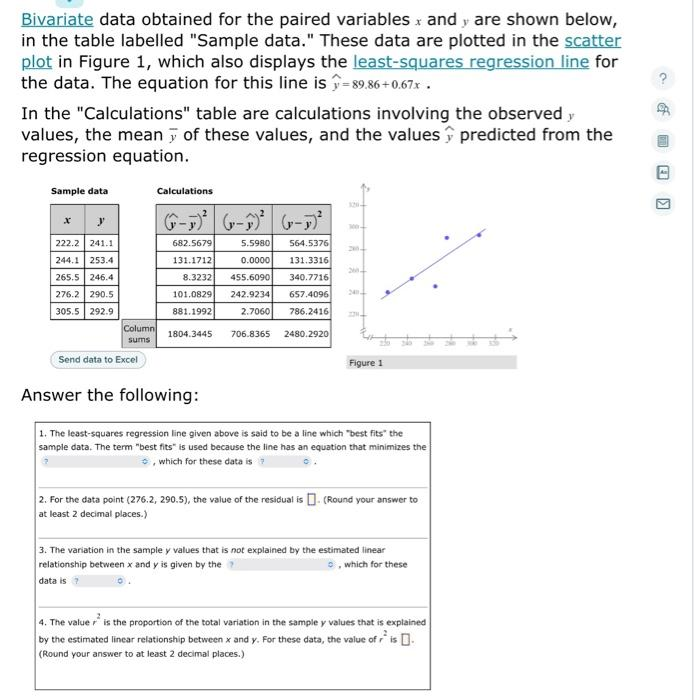# (Solved): Bivariate data obtained for the paired variables $$x$$ and $$y$$ are shown below, in the table ...Bivariate data obtained for the paired variables $$x$$ and $$y$$ are shown below, in the table labelled "Sample data." These data are plotted in the scatter plot in Figure 1, which also displays the least-squares regression line for the data. The equation for this line is $$\hat{y}=89.86+0.67 x$$. In the "Calculations" table are calculations involving the observed $$y$$ values, the mean $$\bar{y}$$ of these values, and the values $$\hat{y}$$ predicted from the regression equation. Sample data Answer the following:

We have an Answer from Expert